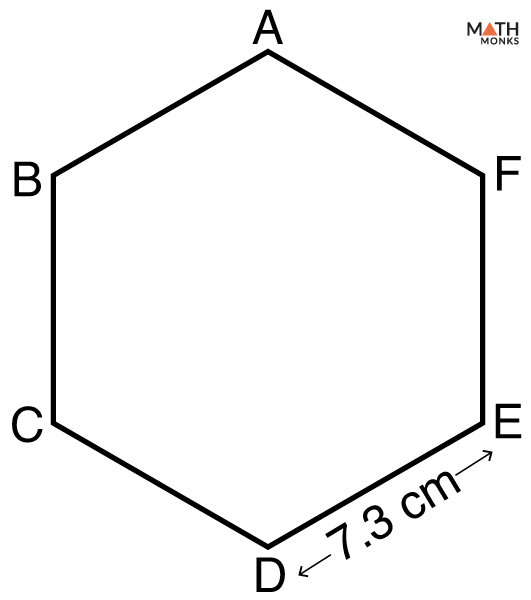# Perimeter of Hexagon

The perimeter of a hexagon is the total distance covered around its edge. The perimeter is always linear. So its units are also linear, such as cm, in, m, ft, or yd.

## Formula

The basic formula to find the perimeter of a regular hexagon is given below:

Let us solve some examples to understand the concept better.

## Solved ExamplesWhat is the perimeter of the hexagon ABCDEF with a side of 7.3 cm?

Solution:

As we know,
Perimeter (P) = 6s, here s = 7.3 cm
= 6 × 7.3
= 43.8 cm

Finding the perimeter of a regular hexagon when AREA is known

Find the perimeter of a hexagon having an area of 54√3 sq. m

Solution:

As we know,
Area (A)  = 3√3/2 × (s)2 , here A = 54√3 sq. m, s = side (unknown)
s2 = (54√3 ÷ 3√3) × 2
= 36
s = 6
Perimeter (P) = 6s, here s = 6 m
= 6 × 6
= 36 m

Finding the perimeter of an irregular hexagon when EACH SIDE is known

Find the perimeter of a hexagon with side lengths 9 cm, 10 cm, 14 cm, 13 cm, 12 cm, and 8 cm.

Solution:

As we know,
Perimeter (P) of an irregular hexagon is the sum of all its sides
P = 9 + 10 + 14 + 13 + 12 + 8
= 66 cm